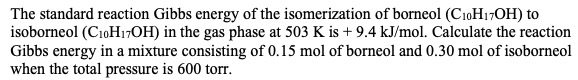# The standard reaction Gibbs energy of the isomerization of borneol (CioH17OH) toisoborneol (C10H7OH) in the gas phase at 503 K is +9.4 kJ/mol. Calculate the reactionGibbs energy in a mixture consisting of 0.15 mol of borneol and 0.30 mol of isoborneolwhen the total pressure is 600 torr

Question
4 viewshelp_outlineImage TranscriptioncloseThe standard reaction Gibbs energy of the isomerization of borneol (CioH17OH) to isoborneol (C10H7OH) in the gas phase at 503 K is +9.4 kJ/mol. Calculate the reaction Gibbs energy in a mixture consisting of 0.15 mol of borneol and 0.30 mol of isoborneol when the total pressure is 600 torr fullscreen
check_circle

Step 1

Borneol --> isoborneol

T= 503 K

R= 8.314 J/K.mole

Total number of moles = 0.15 mole +0.30 mole =0.45 mole

Mole fraction of borneol, Xb = 0.15 mole/ 0.45 mole = 0.33

Mole fraction of isoborneol, Xi = 0.3 mole/ 0.45 mole = 0.66

Partial...

### Want to see the full answer?

See Solution

#### Want to see this answer and more?

Solutions are written by subject experts who are available 24/7. Questions are typically answered within 1 hour.*

See Solution
*Response times may vary by subject and question.
Tagged in

### Chemistry